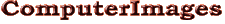Support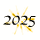# Using Excel’s VLOOKUP Function.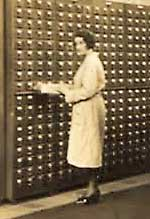Researcher Using Card Catalogue.1

Excel’s VLOOKUP function is like having a reference librarian in your spreadsheet. It takes an input value, looks for it in a reference table and, if it finds it, gives you related data from the table. For example, it can lookup a client’s name from the client’s account number.

First Decide What Type of Lookup You Need. VLOOKUP can make two types of searches: Exact Match, where it seeks an entry identical to the input item; or Range, where it locates the appropriate bracket for the input entry within a list of increasing values. Use Exact Match to find a name connected with an account number — no match is made unless the correct account is found. Use Range lookup to get the tax rate for a certain income level — the IRS table gives the starting point for each rate and VLOOKUP determines which bracket applies to the input salary.

Basic Setup. The lookup process works like a librarian finding something for you. You specify what you are searching for, where to look and what information to retrieve.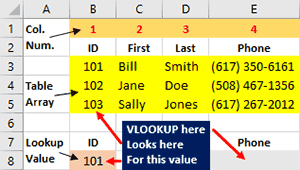VLOOKUP Searches for the Lookup Value in the Leftmost Column of the Table Array.

VLOOKUP requires three pieces of information:

• A “Lookup Value” (what to look for);
• Reference data (where to search). Microsoft calls this the “Table Array;” and
• The column from which to read the result (information to retrieve).

In the example above the Table Array is the yellow cells containing IDs, names and phone numbers. The “V” in VLOOKUP stands for vertical, so the IDs in the Table Array must be listed vertically. Also, the entries to which the Lookup Value is compared must be in the leftmost column of the Table Array.

In the example, the ID in B8 is the Lookup Value and the column designation is part of the formula. The VLOOKUP formula in E8 searches the Table Array for the ID in B8 and gives the associated phone number if a matching ID is found.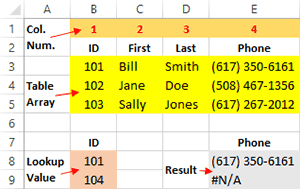Exact Match Looking Up Phone from ID.

Exact Match Lookup. The Exact Match lookup only gives an answer if the Table Array contains an entry identical to the Lookup Value. If it does, the answer is read from a designated cell on the same row as the matching entry. If it does not, the result is “#N/A”.

In our example, the following formula (spaces added for clarity) in E8 looks up the phone number associated with the ID in B8:

=VLOOKUP ( B8 , \$B\$3:\$E\$5 , 4 , FALSE )

B8 specifies the location of the Lookup Value and \$B\$3:\$E\$5 that of the Table Array. The dollar signs in \$B\$3:\$E\$5 prevent those cell references from changing if the formula is autofilled or copied and pasted.

The third entry, “4”, indicates to read the answer from the fourth column of the Table Array (B3:E5). It is important to specify the position of the column within the Table Array, not its location on the worksheet (E).

The fourth entry, “FALSE”, tells Excel not to perform a Range lookup.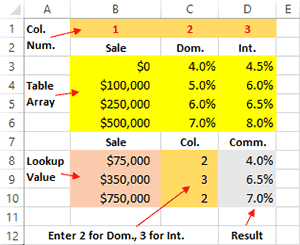Range Lookup Computes Commission Rate from Sale Amount and Type.

Range Look Up. A Range lookup differs from Exact Match in how it searches for the Lookup Value: identifies which interval in a value list the Lookup Value belongs in while Exact Match seeks an identical entry. The example on the right shows a Range lookup being used to determine the commission rate for each sale.

The numbers in column B indicate the dollar level at which each commission rate begins. For example, the rates on Row 3 apply to transactions between \$0 and \$99,999.99. In this case there are different rates for domestic and international sales.

A Range lookup only works if the entries in the leftmost column of the Table Array are listed in ascending order. When comparing the Lookup Value to entries in the Table Array, VLOOKUP starts at the top and goes down. It stops at the last entry that the Lookup Value is greater or equal to. Thus in the example, a \$99,999.99 sale gets the rates on row 3, while a \$100,000.00 sale gets the higher rates on row 4.

The formula (spaces added) in D8 is:

=VLOOKUP ( B8 , \$B\$3:\$D\$6 , C8 , TRUE )

The first two entries provide the location of the Lookup Value and Table Array. The third entry, C8, reads the Column Index Number from from the worksheet so it can be changed without altering the formula (this can be done with Exact Match searches as well). The fourth entry, “TRUE”, makes this a Range lookup.

Use the Function Wizard. The easiest way to insert a function into an Excel formula is the Function Wizard. It provides labeled boxes indicating where the required information should go and explains what is needed.

To use the wizard, click in the cell where you want to insert the function and then click the fx button on either the formula bar or the leftmost side of the Formula ribbon. The animated graphic below demonstrates the process for an Exact Match VLOOKUP.

Troubleshooting. As with any formula, VLOOKUP works correctly only if everything is properly in place. Here are a few things you can try if you are having problems:

• Test your formula on a small data set where you know what the correct answers should be. It can be hard to spot the source of a problem when you are dealing with thousands of rows of information.
• Make sure the Lookup Value doesn't contain hidden characters such as spaces. The Lookup Value “Boston ” will not match to the entry “Boston” in a Table Array.
• Make sure the Column Index Number is correct. Excel will not update it automatically if columns are inserted or deleted within the range of the Table Array.

For additional tools for analyzing formulas, see Getting It Right in Excel.

-----

1. Image from Google Cultural Institute. The catalogue was at the Mundanaeum, an early twentieth century project to create a searchable index of all the world’s information. See Organizing All the World’s Information — Mundaneum.